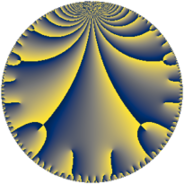Properties

 Label 441.6.a.yLevel $441$ Weight $6$ Character orbit 441.a Self dual yes Analytic conductor $70.729$ Analytic rank $0$ Dimension $4$ CM no Inner twists $4$

Related objects

Newspace parameters

 Level: $$N$$ $$=$$ $$441 = 3^{2} \cdot 7^{2}$$ Weight: $$k$$ $$=$$ $$6$$ Character orbit: $$[\chi]$$ $$=$$ 441.a (trivial)

Newform invariants

 Self dual: yes Analytic conductor: $$70.7292645375$$ Analytic rank: $$0$$ Dimension: $$4$$ Coefficient field: $$\Q(\sqrt{19}, \sqrt{69})$$ Defining polynomial: $$x^{4} - 2 x^{3} - 71 x^{2} + 72 x - 15$$ Coefficient ring: $$\Z[a_1, \ldots, a_{5}]$$ Coefficient ring index: $$2^{8}\cdot 3^{2}$$ Twist minimal: yes Fricke sign: $$-1$$ Sato-Tate group: $\mathrm{SU}(2)$

$q$-expansion

Coefficients of the $$q$$-expansion are expressed in terms of a basis $$1,\beta_1,\beta_2,\beta_3$$ for the coefficient ring described below. We also show the integral $$q$$-expansion of the trace form.

 $$f(q)$$ $$=$$ $$q + \beta_{1} q^{2} + 44 q^{4} + \beta_{2} q^{5} + 12 \beta_{1} q^{8} +O(q^{10})$$ $$q + \beta_{1} q^{2} + 44 q^{4} + \beta_{2} q^{5} + 12 \beta_{1} q^{8} -\beta_{3} q^{10} + 43 \beta_{1} q^{11} -\beta_{3} q^{13} -496 q^{16} -11 \beta_{2} q^{17} + \beta_{3} q^{19} + 44 \beta_{2} q^{20} + 3268 q^{22} + 341 \beta_{1} q^{23} + 6811 q^{25} + 76 \beta_{2} q^{26} + 518 \beta_{1} q^{29} + 11 \beta_{3} q^{31} -880 \beta_{1} q^{32} + 11 \beta_{3} q^{34} -5466 q^{37} -76 \beta_{2} q^{38} -12 \beta_{3} q^{40} + 99 \beta_{2} q^{41} + 12540 q^{43} + 1892 \beta_{1} q^{44} + 25916 q^{46} -100 \beta_{2} q^{47} + 6811 \beta_{1} q^{50} -44 \beta_{3} q^{52} -1738 \beta_{1} q^{53} -43 \beta_{3} q^{55} + 39368 q^{58} + 428 \beta_{2} q^{59} + 55 \beta_{3} q^{61} -836 \beta_{2} q^{62} -51008 q^{64} + 9936 \beta_{1} q^{65} -29996 q^{67} -484 \beta_{2} q^{68} + 7051 \beta_{1} q^{71} + 56 \beta_{3} q^{73} -5466 \beta_{1} q^{74} + 44 \beta_{3} q^{76} + 80168 q^{79} -496 \beta_{2} q^{80} -99 \beta_{3} q^{82} + 308 \beta_{2} q^{83} -109296 q^{85} + 12540 \beta_{1} q^{86} + 39216 q^{88} -209 \beta_{2} q^{89} + 15004 \beta_{1} q^{92} + 100 \beta_{3} q^{94} -9936 \beta_{1} q^{95} + 154 \beta_{3} q^{97} +O(q^{100})$$ $$\operatorname{Tr}(f)(q)$$ $$=$$ $$4 q + 176 q^{4} + O(q^{10})$$ $$4 q + 176 q^{4} - 1984 q^{16} + 13072 q^{22} + 27244 q^{25} - 21864 q^{37} + 50160 q^{43} + 103664 q^{46} + 157472 q^{58} - 204032 q^{64} - 119984 q^{67} + 320672 q^{79} - 437184 q^{85} + 156864 q^{88} + O(q^{100})$$

Basis of coefficient ring in terms of a root $$\nu$$ of $$x^{4} - 2 x^{3} - 71 x^{2} + 72 x - 15$$:

 $$\beta_{0}$$ $$=$$ $$1$$ $$\beta_{1}$$ $$=$$ $$($$$$4 \nu^{3} - 6 \nu^{2} - 294 \nu + 148$$$$)/7$$ $$\beta_{2}$$ $$=$$ $$($$$$48 \nu^{3} - 72 \nu^{2} - 3360 \nu + 1692$$$$)/7$$ $$\beta_{3}$$ $$=$$ $$24 \nu^{2} - 24 \nu - 864$$
 $$1$$ $$=$$ $$\beta_0$$ $$\nu$$ $$=$$ $$($$$$\beta_{2} - 12 \beta_{1} + 12$$$$)/24$$ $$\nu^{2}$$ $$=$$ $$($$$$\beta_{3} + \beta_{2} - 12 \beta_{1} + 876$$$$)/24$$ $$\nu^{3}$$ $$=$$ $$($$$$\beta_{3} + 50 \beta_{2} - 572 \beta_{1} + 872$$$$)/16$$

Embeddings

For each embedding $$\iota_m$$ of the coefficient field, the values $$\iota_m(a_n)$$ are shown below.

For more information on an embedded modular form you can click on its label.

Label $$\iota_m(\nu)$$ $$a_{2}$$ $$a_{3}$$ $$a_{4}$$ $$a_{5}$$ $$a_{6}$$ $$a_{7}$$ $$a_{8}$$ $$a_{9}$$ $$a_{10}$$
1.1
 0.705587 9.01221 −8.01221 0.294413
−8.71780 0 44.0000 −99.6795 0 0 −104.614 0 868.986
1.2 −8.71780 0 44.0000 99.6795 0 0 −104.614 0 −868.986
1.3 8.71780 0 44.0000 −99.6795 0 0 104.614 0 −868.986
1.4 8.71780 0 44.0000 99.6795 0 0 104.614 0 868.986
 $$n$$: e.g. 2-40 or 990-1000 Significant digits: Format: Complex embeddings Normalized embeddings Satake parameters Satake angles

Atkin-Lehner signs

$$p$$ Sign
$$3$$ $$1$$
$$7$$ $$-1$$

Inner twists

Char Parity Ord Mult Type
1.a even 1 1 trivial
3.b odd 2 1 inner
7.b odd 2 1 inner
21.c even 2 1 inner

Twists

By twisting character orbit
Char Parity Ord Mult Type Twist Min Dim
1.a even 1 1 trivial 441.6.a.y 4
3.b odd 2 1 inner 441.6.a.y 4
7.b odd 2 1 inner 441.6.a.y 4
21.c even 2 1 inner 441.6.a.y 4

By twisted newform orbit
Twist Min Dim Char Parity Ord Mult Type
441.6.a.y 4 1.a even 1 1 trivial
441.6.a.y 4 3.b odd 2 1 inner
441.6.a.y 4 7.b odd 2 1 inner
441.6.a.y 4 21.c even 2 1 inner

Hecke kernels

This newform subspace can be constructed as the intersection of the kernels of the following linear operators acting on $$S_{6}^{\mathrm{new}}(\Gamma_0(441))$$:

 $$T_{2}^{2} - 76$$ $$T_{5}^{2} - 9936$$ $$T_{13}^{2} - 755136$$

Hecke characteristic polynomials

$p$ $F_p(T)$
$2$ $$( -76 + T^{2} )^{2}$$
$3$ $$T^{4}$$
$5$ $$( -9936 + T^{2} )^{2}$$
$7$ $$T^{4}$$
$11$ $$( -140524 + T^{2} )^{2}$$
$13$ $$( -755136 + T^{2} )^{2}$$
$17$ $$( -1202256 + T^{2} )^{2}$$
$19$ $$( -755136 + T^{2} )^{2}$$
$23$ $$( -8837356 + T^{2} )^{2}$$
$29$ $$( -20392624 + T^{2} )^{2}$$
$31$ $$( -91371456 + T^{2} )^{2}$$
$37$ $$( 5466 + T )^{4}$$
$41$ $$( -97382736 + T^{2} )^{2}$$
$43$ $$( -12540 + T )^{4}$$
$47$ $$( -99360000 + T^{2} )^{2}$$
$53$ $$( -229568944 + T^{2} )^{2}$$
$59$ $$( -1820116224 + T^{2} )^{2}$$
$61$ $$( -2284286400 + T^{2} )^{2}$$
$67$ $$( 29996 + T )^{4}$$
$71$ $$( -3778461676 + T^{2} )^{2}$$
$73$ $$( -2368106496 + T^{2} )^{2}$$
$79$ $$( -80168 + T )^{4}$$
$83$ $$( -942568704 + T^{2} )^{2}$$
$89$ $$( -434014416 + T^{2} )^{2}$$
$97$ $$( -17908805376 + T^{2} )^{2}$$